# NCERT Solutions for Class 8 Maths Chapter 7 Cubes and Cube Roots| PDF Download

Studyrankers subject matter experts have designed Chapter 7 Cubes and Cube Roots NCERT Solutions for Class 8 Maths in such a way that will help you in improving the marks in the examinations and have edge over your classmates. You can download PDF of Class 9 Maths Chapter 15 Probability NCERT Solutions make you able to solve the difficult problems in a exercise. Chapter 7 NCERT Solutions help in solving the difficulties that lie ahead with ease.

NCERT Solutions for Class 8 Maths which will develop you understanding of the chapter and obtain maximum marks in the exams. You can also complete your homework on time through the help of these Chapter 7 Cubes and Cube Roots NCERT Solutions and able to solve the difficult problems in a given in a exercise.Page No: 114

Exercise 7.1

1. Which of the following numbers are not perfect cubes?
(i) 216

Solution

By resolving 216 into prime factor,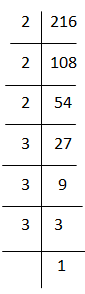216 = 2×2×2×3×3×3
By grouping the factors in triplets of equal factors,
216 = (2×2×2)×(3×3×3)
Here, 216 can be grouped into triplets of equal factors,
∴ 216 = (2×3) = 6

Hence, 216 is cube of 6.

(ii)  128

Solution

By resolving 128 into prime factor,128 = 2×2×2×2×2×2×2
By grouping the factors in triplets of equal factors,
128 = (2×2×2)×(2×2×2)×2
Here, 128 cannot be grouped into triplets of equal factors, we are left of with one factors 2 .
∴ 128 is not a perfect cube.

(iii) 1000

Solution

By resolving 1000 into prime factor,1000 = 2×2×2×5×5×5
By grouping the factors in triplets of equal factors,
1000 = (2×2×2)×(5×5×5)
Here, 1000 can be grouped into triplets of equal factors,
∴ 1000 = (2×5) = 10
Hence, 1000 is cube of 10.

(iv) 100

Solution

By resolving 100 into prime factor,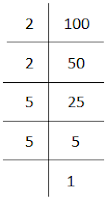100 = 2×2×5×5
Here, 100 cannot be grouped into triplets of equal factors.
∴ 100 is not a perfect cube.

(v)  46656

Solution

By resolving 46656 into prime factor,46656 = 2×2×2×2×2×2×3×3×3×3×3×3
By grouping the factors in triplets of equal factors,
46656 = (2×2×2)×(2×2×2)×(3×3×3)×(3×3×3)
Here, 46656 can be grouped into triplets of equal factors,
∴ 46656 = (2×2×3×3) = 36
Hence, 46656 is cube of 36.

2.Find the smallest number by which each of the following numbers must be multiplied to obtain a perfect cube.

(i) 243

Solution

By resolving 243 into prime factor,243 = 3×3×3×3×3
By grouping the factors in triplets of equal factors,
243 = (3×3×3)×3×3
Here, 3 cannot be grouped into triplets of equal factors.
∴ We will multiply 243 by 3 to get perfect square.

(ii) 256

Soliution

By resolving 256 into prime factor,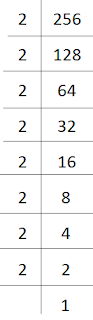256 = 2×2×2×2×2×2×2×2
By grouping the factors in triplets of equal factors,
256 = (2×2×2)×(2×2×2)×2×2
Here, 2 cannot be grouped into triplets of equal factors.
∴ We will multiply 256 by 2 to get perfect square.

(iii) 72

Solution

By resolving 72 into prime factor,72 = 2×2×2×3×3
By grouping the factors in triplets of equal factors,
72 = (2×2×2)×3×3
Here, 3 cannot be grouped into triplets of equal factors.
∴ We will multiply 72 by 3 to get perfect square.

(iv)  675

Solution

By resolving 675 into prime factor,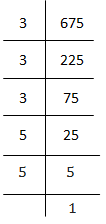675 = 3×3×3×5×5
By grouping the factors in triplets of equal factors,
675 = (3×3×3)×5×5
Here, 5 cannot be grouped into triplets of equal factors.
∴ We will multiply 675 by 5 to get perfect square.

(v) 100

Solution

By resolving 100 into prime factor,100 = 2×2×5×5
Here, 2 and 5  cannot be grouped into triplets of equal factors.
∴ We will multiply 100 by (2×5) 10 to get perfect square.

3.Find the smallest number by which each of the following numbers must be divided to obtain a perfect cube.

(i) 81

Solution

By resolving 81 into prime factor,81 = 3×3×3×3
By grouping the factors in triplets of equal factors,
81 = (3×3×3)×3
Here, 3 cannot be grouped into triplets of equal factors.
∴ We will divide 81 by 3 to get perfect square.

(ii) 128

Solution

By resolving 128 into prime factor,128 = 2×2×2×2×2×2×2
By grouping the factors in triplets of equal factors,
128 = (2×2×2)×(2×2×2)×2
Here, 2 cannot be grouped into triplets of equal factors.
∴ We will divide 128 by 2 to get perfect square.

(iii) 135

Solution

By resolving 135 into prime factor,135 = 3×3×3×5
By grouping the factors in triplets of equal factors,
135 = (3×3×3)×5
Here, 5 cannot be grouped into triplets of equal factors.
∴ We will divide 135 by 5 to get perfect square.

(iv) 192

Solution

By resolving 192 into prime factor,192 = 2×2×2×2×2×2×3
By grouping the factors in triplets of equal factors,
192 = (2×2×2)×(2×2×2)×3
Here, 3 cannot be grouped into triplets of equal factors.
∴ We will divide 192 by 3 to get perfect square.

(v)  704

Solution

By resolving 704 into prime factor,704 = 2×2×2×2×2×2×11
By grouping the factors in triplets of equal factors,
704 = (2×2×2)×(2×2×2)×11
Here, 11 cannot be grouped into triplets of equal factors.
∴ We will divide 704 by 11 to get perfect square.

4. Parikshit makes a cuboid of plasticine of sides 5 cm, 2 cm, 5 cm. How many such cuboids will he need to form a cube?

Solution

Given, side of cube is 5 cm, 2 cm and 5 cm.
∴ Volume of cube = 5×2×5 = 5050 = 2×5×5
Here, 2 , 5 and 5 cannot be grouped into triplets of equal factors.
∴ We will multiply 50 by (2×2×5) 20  to get perfect square.
Hence, 20 cuboid is needed.

Page No. 116

Exercise 7.2

1. Find the cube root of each of the following numbers by prime factorisation method
(i) 64

Solution

64 = 2×2×2×2×2×2
By grouping the factors in triplets of equal factors,
64 = (2×2×2)×(2×2×2)
Here, 64 can be grouped into triplets of equal factors,
∴ 64 = 2×2 = 4
Hence, 4 is cube root of 64.

(ii) 512

Solution

512 = 2×2×2×2×2×2×2×2×2
By grouping the factors in triplets of equal factors,
512 = (2×2×2)×(2×2×2)×(2×2×2)
Here, 512 can be grouped into triplets of equal factors,
∴ 512 = 2×2×2 = 8
Hence, 8 is cube root of 512.

(iii) 10648

Solution

10648 = 2×2×2×11×11×11
By grouping the factors in triplets of equal factors,
10648 = (2×2×2)×(11×11×11)
Here, 10648 can be grouped into triplets of equal factors,
∴ 10648 = 2 ×11 = 22
Hence, 22 is cube root of 10648.

(iv) 27000

Solution

27000 = 2×2×2×3×3×3×3×5×5×5
By grouping the factors in triplets of equal factors,
27000 = (2×2×2)×(3×3×3)×(5×5×5)
Here, 27000 can be grouped into triplets of equal factors,
∴ 27000 = (2×3×5) = 30
Hence, 30 is cube root of 27000.

(v) 15625

Solution

15625 = 5×5×5×5×5×5
By grouping the factors in triplets of equal factors,
15625 = (5×5×5)×(5×5×5)
Here, 15625 can be grouped into triplets of equal factors,
∴ 15625 = (5×5) = 25
Hence, 25 is cube root of 15625.

(vi) 13824

Solution

13824 = 2×2×2×2×2×2×2×2×2×3×3×3
By grouping the factors in triplets of equal factors,
13824 = (2×2×2)×(2×2×2)×(2×2×2)×(3×3×3)
Here, 13824 can be grouped into triplets of equal factors,
∴ 13824 = (2×2× 2×3) = 24
Hence, 24 is cube root of 13824.

(vii) 110592

Solution

110592 = 2×2×2×2×2×2×2×2×2×2×2×2×3×3×3
By grouping the factors in triplets of equal factors,
110592 = (2×2×2)×(2×2×2)×(2×2×2)×(2×2×2)×(3×3×3)
Here, 110592 can be grouped into triplets of equal factors,
∴ 110592 = (2×2×2×2 × 3) = 48
Hence, 48 is cube root of 110592.

(viii) 46656

Solution

46656 = 2×2×2×2×2×2×3×3×3×3×3×3
By grouping the factors in triplets of equal factors,
46656 = (2×2×2)×(2×2×2)×(3×3×3)×(3×3×3)
Here, 46656 can be grouped into triplets of equal factors,
∴ 46656 = (2×2×3×3) = 36
Hence, 36 is cube root of 46656.

(ix) 175616

Solution

175616 = 2×2×2×2×2×2×2×2×2×7×7×7
By grouping the factors in triplets of equal factors,
175616 = (2×2×2)×(2×2×2)×(2×2×2)×(7×7×7)
Here, 175616 can be grouped into triplets of equal factors,
∴ 175616 = (2×2×2×7) = 56
Hence, 56 is cube root of 175616.

(x) 91125

Solution

91125 = 3×3×3×3×3×3×3×5×5×5
By grouping the factors in triplets of equal factors,
91125 = (3×3×3)×(3×3×3)×(5×5×5)
Here, 91125 can be grouped into triplets of equal factors,
∴ 91125 = (3×3×5) = 45
Hence, 45 is cube root of 91125.

2.State true or false.
(i) Cube of any odd number is even.

Ans.False

(ii) A perfect cube does not end with two zeros.

Ans.True

(iii) If square of a number ends with 5, then its cube ends with 25.

Ans.False

(iv) There is no perfect cube which ends with 8.

Ans.False

(v) The cube of a two digit number may be a three digit number.

Ans.False

(vi) The cube of a two digit number may have seven or more digits.

Ans.False

(vii) The cube of a single digit number may be a single digit number.

Ans.True

4. You are told that 1,331 is a perfect cube. Can you guess without factorisation what is its cube root? Similarly, guess the cube roots of 4913, 12167, 32768.

Solution

• By grouping the digits, we get 1 and 331
As we know that the unit’s digit of the cube of a number having digit as unit’s place 1 is 1.
∴ We get 1 as unit digit of the cube root of 1331.
The cube of 1 matches with the number of second group.
∴ The ten digit of our cube root is taken as the unit place of smallest number.
As we know that the unit’s digit of the cube of a number having digit as unit’s place 1 is 1.
∴= 11

• By grouping the digits, we get 4 and 913
As we know that the unit’s digit of the cube of a number having digit as unit’s place 3 is 7.
∴ we get 7 as unit digit of the cube root of 4913.
We know 1= 1  and 2 = 8 , 1 > 4 > 8.
Thus, 1 is taken as ten digit of  cube root.
∴= 17

• By grouping the digits, we get 12 and 167.
As we know that the unit’s digit of the cube of a number having digit as unit’s place 7 is 3.
∴ we get 3 as unit digit of the cube root of 12167
We know 2= 8  and 3 = 27 , 8 > 12 > 27.
Thus, 2 is taken as ten digit of  cube root.
∴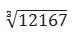= 23

• By grouping the digits, we get 32 and 768.
As we know that the unit’s digit of the cube of a number having digit as unit’s place 8 is 2.
∴ we get 2 as unit digit of the cube root of 32768.
We know 33 = 27  and 43 = 64 , 27 > 32 > 64.
Thus,  3 is taken as ten digit of  cube root.
∴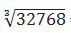= 32

## NCERT Solutions for Class 8 Maths Chapter 7 Cubes and Cube Roots

Chapter 7  Class 8 Maths NCERT Solutions is going to help you a lot in understand about cube roots. If we multiply a number by itself three times, the product so obtained is called the perfect cube of that number.

• Cubes of even numbers are even and those of odd numbers are odd.

• There are only 10 perfect cubes from 1 to 1000.

• The cube of a negative number is always negative.

• If the digit in the one’s place of a number is 0, 1, 4, 5, 6 or 9, then its cube will end in the same digit.

• If the digit in the one’s place of a number is 2, then the ending digit of its cube will be 8 and vice-versa.

• If the digit in the one’s place of a number is 3, then the ending digit of its cube will be 7 and vice-versa.

• If the prime factors of a number cannot be made into groups of 3, it is not a perfect cube.

There are only two exercises in the whole Chapter Cubes and Cube Roots which you need to solve to develop basic concepts. These NCERT Solutions  are updated according to the latest NCERT Class 8 Maths textbook and syllabus.

NCERT Solutions for Class 8 Maths that are updated as per the latest marking scheme and syllabus prescribed by CBSE.

### NCERT Solutions for Class 8 Maths Chapters:

 Chapter 1 Rational Numbers Chapter 2 Linear Equations in Variable Chapter 3 Understanding Quadrilaterals Chapter 4 Practical Geometry Chapter 5 Data Handling Chapter 6 Squares and Square Roots Chapter 8 Comparing Quantities Chapter 9 Algebraic Expressions and Identities Chapter 10 Visualizing Solid Shapes Chapter 11 Mensuration Chapter 12 Exponents and Powers Chapter 13 Direct and Inverse Proportions Chapter 14 Factorization Chapter 15 Introduction to Graphs Chapter 16 Playing with Numbers

FAQ on Chapter 7 Cubes and Cube Roots

#### What are the benefits of NCERT Solutions for Chapter 7 Cubes and Cube Roots Class 8 NCERT Solutions?

Chapter 7 Class 8 Maths NCERT Solutions will develop your problem solving skills and make aware of the difficulty of questions. These NCERT Solutions will serve as beneficial tool that can be used to recall various questions any time.

#### Write any two properties of Perfect Cubes?

If the digit in the one’s place of a number is 2, the digit in the one’s place of its cube is 8, and vice-versa and If the digit in the one’s place of a number is 0, 1, 4, 5, 6 or 9, then the digit in the one’s place of its cube will also be the same digit.

#### Is 31944 a perfect cube? If not then by which smallest natural number should 31944 be divided so that the quotient is a perfect cube?

We have 31944 = 2 × 2 × 2 × 3 × 11 × 11 × 11
Since, the prime factors of 31944 do not appear in triples as 3 is left over.
∴ 31944 is not a perfect cube. Obviously, 31944 / 3 will be a perfect cube
i.e.  ÷3 = [2 × 2 × 2 × 3 × 11 × 11 × 11] /3
or
10648 = 2 × 2 × 2 × 11 × 11 × 11
∴ 10648 is a perfect cube.
Thus, the required least number = 3.

#### Is 500 a perfect cube?

500 = 5 × 5 × 5 × 2 × 2
∵ In the  prime factorisation 2 × 2 remain after grouping the prime factors in triples.
∴ 500 is not a perfect cube.